# Class 10 Science Chapter 10 Light- Reflection and Refraction Study Notes

### Properties of Light

• Electromagnetic wave, so does not require any medium to travel.
• Light tends to travel in straight line.
• Light has dual nature i.e., wave as well as particle.
• Speed of light is maximum in vacuum. Its value is 3 × 10^8 m/s.
• When light falls on a surface, following may happen :
(a) Reflection
(b) Refraction
(c) Absorption

### REFLECTION

Bouncing back of light when it strikes on a polished surface like mirror.

### Laws of Reflection :

(1) Angle of incidence is equal to the angle of reflection.
(2) The incident ray, the reflected ray and the normal at the point of incidence, all lie in the same plane.### Image :

It is a point where atleast two light rays actually meet or appear to meet.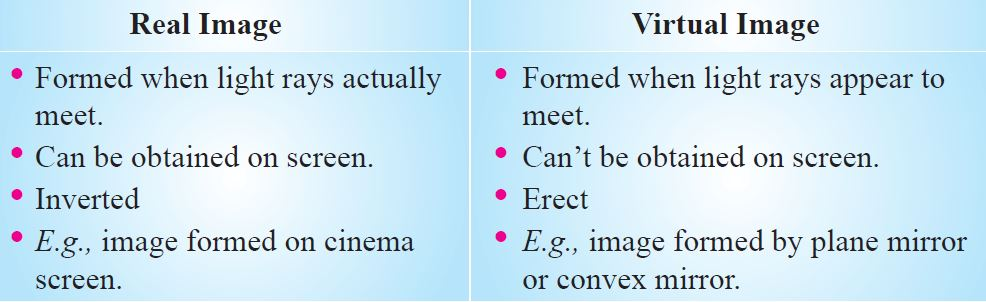### Image Formed by Plane Mirror:### Characteristics of Image:

(i) Virtual and erect.
(ii) Size of image is equal to the size of object.
(iii) Image is formed as far behind the mirror as the object is in front of it.
(iv) Laterally inverted.

### Lateral Inversion :

The right side of the object appears left side of the image and vice-versa.

### Application of lateral inversion :

The word AMBULANCE is written as so that it can be read correctly in rear view mirror of vehicles going in front of it.

Spherical Mirrors : Mirrors whose reflecting surface is curved.For Convex Mirror:

• Reflecting surface is curved outwards.

• Diverging mirror

For Concave Mirror:

• Reflecting surface is curved inwards.

• Converging mirror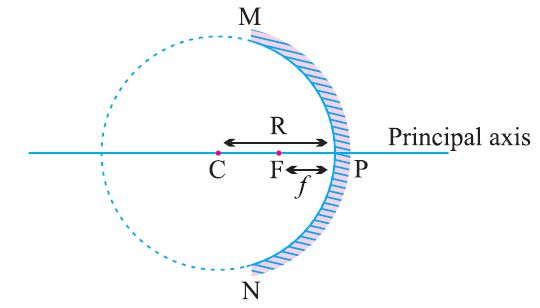• Principal axis : The line joining the pole and center of curvature.
• Pole (P) : The centre of the spherical mirror.
• Aperture (MN) : It is the effective diameter of the spherical mirror.
• Center of Curvature (C) : The centre of the hollow glass sphere of which the mirror was a part.
• Radius of Curvature (R) : The distance between the pole and the centre of curvature.
• Focus (F) : The point on principal axis where all the parallel light rays actually meet or appear to meet after reflection.
• Focal length (f) : The distance between the pole and the focus.

## f=R/2

### Rules for making ray diagrams by concave mirror:

(i) A ray parallel to the principal axis will pass through the principal focus, after reflection.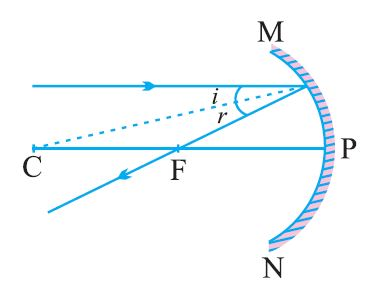(ii) A ray passing through the principal focus of concave mirror will emerge parallel to principal axis after reflection.(iii) A ray of light passing through the centre of curvature of a concave mirror is reflected back along the same path as it is a normally incident ray.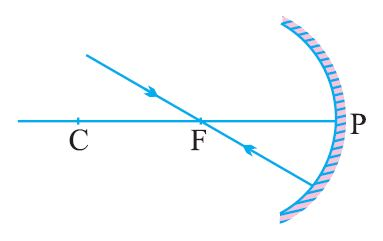(iv) A ray incident obliquely to the principal axis of a concave mirror is reflected obliquely making equal angle.### Ray diagrams for images formed by concave mirror

(i) When object is at infinity :Image
Position − At ‘F’
Nature – Real, inverted
Size – Point sized or highly
diminished

(ii) When object is beyond ‘C’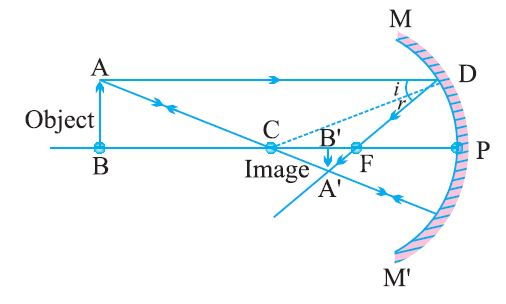Image
Position – Between ‘F’ and ‘C’
Nature – Real, inverted
Size – Diminished

(iii) When object is at ‘C’Image
Position – At ‘C’
Nature – Real, inverted
Size – Same size as that of
object

(iv) When object is placed between ‘F’ and ‘C’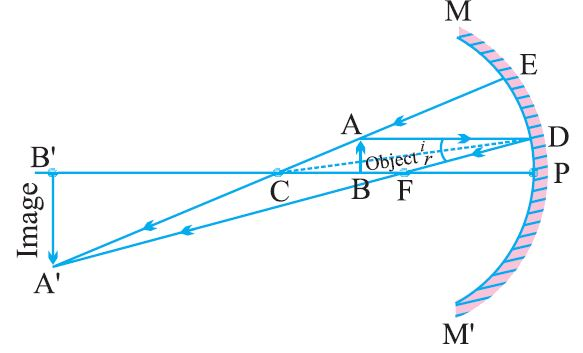Image
Position – Beyond ‘C’
Nature – Real, inverted
Size – Enlarged

(v) When object is placed at ‘F’Image
Position – At Infinity
Nature – Real, inverted
Size – Highly enlarged

(vi) When object is between ‘P’ and ‘F’Image

Position – Behind the mirror
Nature – Virtual, erect
Size – Enlarged

### Uses of Concave Mirror:

(i) Used in torches, search lights and vehicles headlights to get powerful
parallel beam of light.(ii) Concave mirrors are used by dentists to see large image of teeth of
patients. (Teeth have to be placed between pole and focus).(iii) Concave mirror is used as shaving mirror to see a larger image of the
face.
(iv) Large concave mirrors are used to concentrate sunlight to produce heat
in solar furnace.

### Rule for image formation by Convex Mirror:

(i) A ray of light parallel to the principal axis of a convex mirror appear to diverge from the principal focus.(ii) A ray which is directed towards the focus of the convex mirror will emerge parallel to the principal axis after reflection.(iii) A ray directed towards the center of curvature of a convex mirror is reflected back along the same.(iv) A ray incident obliquely to the principal axis is reflected obliquely.### Ray diagrams of images formed by convex mirror:

(i) When object is placed at infinity :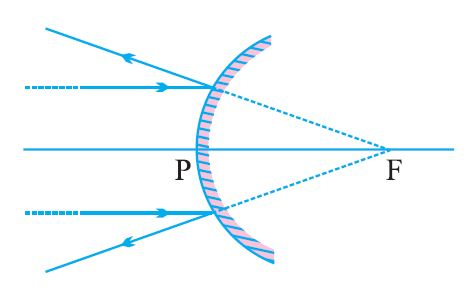Image
Position − At ‘F’
Nature – Virtual, erect
Size – Point sized

(ii) When object is placed between pole and infinityImage
Position – Between ‘P’ and ‘F’
Nature – Virtual, erect
Size – Diminished

• A full length image of a tall building/tree can be seen in a small convex mirror.

### Uses of Convex Mirror:

(i) Convex mirrors are used as rear view mirrors in vehicles because

(a) they always give an erect though diminished image.
(b) they have a wider field of view as they are curved outwards.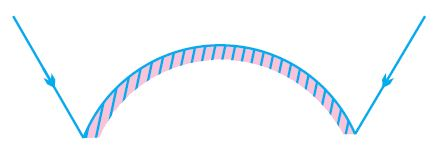(ii) Convex mirrors are used at blind turns and on points of merging traffic to facilitate vision of both side traffic.
(iii) Used in shops as security mirror.

### Sign Convention for Reflection by Spherical MirrorOrNew Cartesian Sign Convention

(i) The object is placed to the left of the mirror.

(ii) All distances parallel to the principal axis are measured from the pole of the mirror.

(iii) All distances measured in the direction of incident ray (along + X-axis) are taken as positive and those measured against the direction of incident ray (along – X-axis) are taken as negative.

(iv) Distance measured perpendicular to and above the principal axis are taken as positive.

(v) Distances measured perpendicular to and below the principal axis are taken as negative.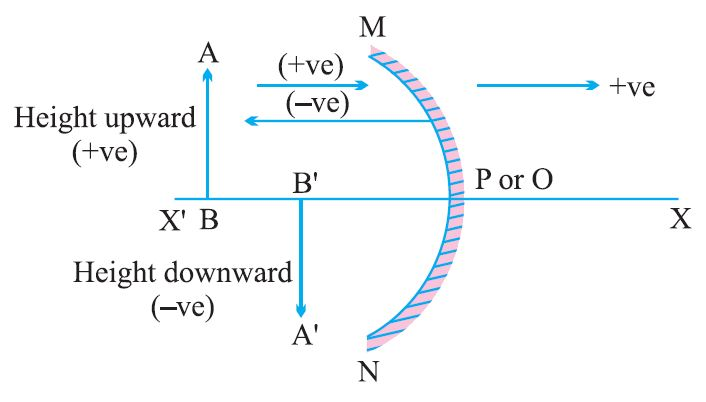• Object distance = ‘u’ is always negative.
• Focal length of concave mirror = Negative
• Focal length of convex mirror = Positive

### Mirror Formula :### Magnification of Spherical Mirrors:

It is the ratio of height of the image to the height of the object.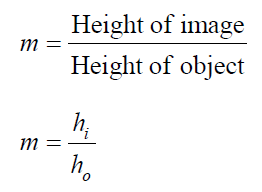Also, m = – v/u

• If ‘m’ is negative, image is real.
• If ‘m’ is positive, image is virtual.
• If hi = ho then m = 1, i.e., image is equal to object.
• If hi > hi then m > 1 i.e., image is enlarged.
• If hi < ho then m < 1 i.e., image is diminished.

Note:

(1) Magnification of plane mirror is always + 1.

‘1’ indicates that image is equal to object’s size.

(2) If ‘m’ is ‘+ve’ and less than 1, it is a convex mirror.

(3) If ‘m’ is ‘+ve’ and more than 1, it is a concave mirror.

(4) If ‘m’ is ‘-ve’, it is a concave mirror.

1. Magnification of plane mirror is + 1. What does it indicate ?

2. A real image, 1/5th size of object is formed at a distance of 18 cm from a mirror. What is the nature of the mirror ? Calculate its focal length.

3. Name the type of mirror used in the following and reason for using it :
(a) Solar furnace
(b) Rear view mirror in a vehicle

4. What should be the position of the object, when a concave mirror is used :
(a) as a shaving mirror ?
(b) in torches as reflecting mirror ?

5. (a) Define principal focus of a spherical mirror.
(b) For what position of the object does a concave mirror form a real, inverted and   diminished image of the object ? Draw the ray diagram.
(c) An object 4 cm high is placed at a distance of 6 cm in front of a concave mirror of focal length 12 cm. Find the position of the image.

6. For what position of an object, a concave mirror forms a real image equal to size of object ?

7. Identify the nature of mirror and mention two characteristics of image formed when magnification m = + 6.

8. Suggest a method to find approximate focal length of a concave mirror.

9. Draw ray diagram when :
(a) object is placed between pole and focus of a concave mirror.
(b) object is placed at infinity from a convex mirror.

10. Name the type of spherical mirror which
(a) has positive focal length.
(b) always forms a virtual image.

# REFRACTION

Bending of light when it enters obliquely from one transparent medium to another.

Speed of light is maximum in vacuum. It is 3 × 10^8 m/s.
• Cause of refraction : Change in speed of light.Some examples of refraction :

(i) The bottom of swimming pool appears higher.

(ii) A pencil partially immersed in water appears to be bent at the interface of water and air.(iii) Lemons placed in a glass tumbler appear bigger.

(iv) Letters of a book appear to be raised when seen through a glass slab.

### Refraction through glass slab:The extent of bending of ray of light at the opposite parallel faces of rectangular glass slab is equal and opposite, so the ray emerges parallel to incident ray.Lateral displacement depends on : (a) Refractive index of glass slab  (b) Thickness of the glass slab

### Laws of Refraction:

(i) The incident ray, the refracted ray and the normal to the interface of two transparent media at the point of incidence, all lie in the same plane.

(ii) Snell’s law : The ratio of sine of angle of incidence to the sine of angle of refraction is a constant, for a light of given colour and for a given pair of media.Refractive index (n) : The ratio of speed of light in vaccum or air to the speed of light in a medium is called refractive index.

Announcements

Join our Online JEE Test Series for 499/- Only (Web + App) for 1 Year

Join our Online NEET Test Series for 499/- Only for 1 Year